# Optimum Design of Fractional Order PIᵅ Speed Controller for Predictive Direct Torque Control of a Sensorless Five-Phase Permanent Magnet Synchronous Machine (PMSM)

Optimum Design of Fractional Order PIᵅ Speed Controller for Predictive Direct Torque Control of a Sensorless Five-Phase Permanent Magnet Synchronous Machine (PMSM)
Laboratoire de Génie Electrique, Faculté de Technologie, Université de M'sila, M'sila 28000, Algeria

Corresponding Author Email:
noureddine.bounasla@univ-msila.dz
Page:
437-449
|
DOI:
https://doi.org/10.18280/jesa.530401
10 June 2020
|
Accepted:
1 August 2020
|
Published:
30 September 2020
| Citation

OPEN ACCESS

Abstract:

In both direct torque control (DTC) and predictive direct torque control (PDTC) strategies, just single voltage vector is applied. The question arose, is this applied vector the optimumin terms of minimizing torque and stator flux ripples? In DTC, it may not be the optimum one. However, in case of PDTC, there is a possibility to evaluate the performance of different voltage vectors, where a cost function is proposed to determine the appropriate voltage vector that brings the lowest torque and stator flux ripple within one cycle. On the other hand, PI controller provides a good performance but if the parameters change, the system may lose its performance. With the aim of enhancing the robustness of the PDTC scheme, a fractional order PI controller is proposed that can be considered as a generalization of the classical PI controller, and to set its parameters, a Grey Wolf Optimization algorithm is employed. Furthermore, omitting the sensor increases reliability and decrease the size and cost of the drive system. For these reasons, an extended Kalman filter observer is adopted, where the rotor speed and rotor position as well as the load torque are estimated. In this work, a fractional order PI controller tuned by GWO for PDTC of a five-phase permanent magnet synchronous machine (PMSM) based on EKF observer is presented. Analysis of simulation results exhibit clearly the efficiency and robustness of the suggested control compared to conventional DTC based on classical PI controller.

Keywords:

five-phase PMSM, DTC, PDTC, fractional order PI controller, grey wolf optimization algorithm, extended Kalman filter

1. Introduction

Compared to three-phase machines, the usage of multiphase machines allows to obtain less torque ripples, can produce higher torque per phase current, and guarantee a reliable drive that keep working with a failure in one or more phases. Due to these merits, this has motivated researchers to increase interest in the use of multiphase machine for critical applications such as electrical propulsion systems for ships, offshore wind farms, and electric aircraft. Among the multiphase machines, five-phase and six phase induction or synchronous machines are the most considered in the literature [1-4]. In the present study a special focus will be given to the five-phase permanent magnet synchronous motor drive (PMSM).

In order to ensure an effective control of five-phase PMSM, various control methods have been suggested in the literature. Although these control methods have a different principle, but it leads to achieve the same main goal consisting of decoupled control between flux and torque, similar to a DC machine with separate excitation. One of these strategies is the direct torque control [5-9]. It has a simple control structure, because it does not require the use of inner current control loops, pulse width modulation block and less parameters dependence; this leads to give a good dynamic performance compared to vector control. However, the use of hysteresis controllers generates a variable switching frequency that leads to the emergence of high ripples in the torque and stator flux. This latter drawback is the major disadvantage of the conventional DTC. This shortcoming can be effectively overcome by using a space vector modulation (SVM)algorithm. Indeed, instead of a switching table and hysteresis controllers, an SVM with linear PI controllers are used. The combination of conventional DTC and SVM forms the direct torque control space vector modulation (DTC-SVM) [10-13]. However, in spite of the mentioned advantage, the DTC-SVM dynamic still depends on the quality of the applied PI controller design algorithm.

Other structure of the DTC based on predictive approach known as Model Predictive Control (MPC) has been lately published in the domain of electrical drives [14-20]. This control strategy manages to minimize torque and stator flux ripples. It can be divided into two main classes, namely, continuous and finite-state model predictive control. The continuous MPC (C-MPC), it can give good performance. However, the use of modulator block increases the complexity of control design. On the contrary, finite-state MPC (FS-MPC) does not need to use a modulation block, it integrates the converters model in the control design. In the literature, the main FS-MPC strategies used to control electrical drives can be divided into two distinct categories:(i) predictive current control (PCC) and (ii) predictive torque control (PTC), which will be the focus of our attention in this paper. it relies on to apply an only single control vector during a sampling period. From this point, it is similar to DTC using a switching table that may not be the optimum one in terms of minimizing torque and stator flux ripples. In the contrary of the DTC, the PDTC examines the impact of each one and selects one that reduces the value of cost function, which defines the behavior of the system.

The PDTC strategy for five-phase PMSM adopts on classical PI controller can achieve a good performance, but it may fail when the parameters change or in presence of external disturbance. In order to face these drawbacks, a fractional order PI controller method is proposed [21-25]. Fractional order PI controller, suggested by Podlubny , is considered as a generalization of the classical PI controller. It can enhance the robustness of the PDTC scheme, and gives good performance results compared to classical PI controller thanks to its extra real parameters implying a greater flexibility. However, it is not an easy task to determine the parameters that can give good results; manual tuning may be a stressful and difficult task even for expert users. To deal with this difficulty, many research works have been focused on this problem. They can be divided into analytical, numerical, rule-based, self-tuning, and evolutionary algorithms optimization methods [27-31].

Grey wolf optimizer (GWO) algorithm is newly innovated ; it emulates the leadership hierarchy and hunting behavior of grey wolves in nature, it has been applied and has been proven effective in several optimization problems [33-41]. Herein, GWO algorithm is used to tune and get the optimal values for the parameters of fractional order PI controller through the minimization of the Integral Time Absolute Error (ITAE) criteria.

To achieve a good speed tracking, an accurate knowledge of rotor shaft position and speed is required. This goal is usually fulfilled by using an external dedicated sensor. However, the use of a sensor leads to an increase in the cost, weight, volume, restricts the application area, and reduces the motor reliability. To solve this issue, sensorless control technology is used to avoid using mechanical sensors. A lot of speed observers have been proposed in the literature [42-48]. In this work, a simple approach based on extended Kalman filter (EKF) is adopted, where the rotor speed and rotor position as well as the load torque are estimated, this observer is known by its rapid and accurate estimation .

The main purpose of this paper is to improve the DTC performance of a five-phase PMSM drive. This objective is accomplished via the use of: (1) PDTC to overcome most of conventional DTC disadvantages, (2) fractional order PI controller tuned by GWO algorithm to enhance the robustness of the PDTC scheme, and (3) EKF to estimate the rotor speed and position as well as the load torque, in order to increase the reliability of the system and to decrease its cost.

The structure of this paper is organized as follows: In Section 2, a mathematical model of the five-phase PMSM is presented. Steps to apply the Extended Kalman Filter are detailed in Section 3. Conventional DTC using large voltage vectors only based on EKF observer is exhibited in Section 4. In Section 5, PDTC strategy based on EKF observer with fractional order PI speed controller optimized by GWO is presented. The simulation results are illustrated and discussed in Section 6. Finally, Section 7 concludes the paper.

2. Five-Phase PMSM Modeling

The model of the five-phase PMSM is totally defined in a (d-q) reference frame through its electrical, magnetic, and mechanical equations [9, 49]:

The stator voltages are as follows:

$\left\{\begin{array}{l}V_{d}=R_{s} i_{d}+\frac{d \phi_{d}}{d t}-\omega_{r} \phi_{q} \\ V_{q}=R_{s} i_{q}+\frac{d \phi_{q}}{d t}+\omega_{r} \phi_{d}\end{array}\right.$      (1)

- The stator flux components in the (d-q) frame is given by:

\left\{ \begin{align}& {{\phi }_{d}}={{L}_{d}}{{i}_{d}}+{{\phi }_{f}} \\& {{\phi }_{q}}={{L}_{q}}{{i}_{q}} \\\end{align} \right.   (2)

- The mechanical dynamic and electromagnetic torque equations are expressed by:

\left\{ \begin{align}& \frac{d{{\omega }_{r}}}{dt}=\frac{1}{J}\text{ }\left( {{T}_{em}}-{{T}_{L}}-f{{\omega }_{r}} \right) \\& \text{ }{{T}_{em}}=\frac{5}{2}p({{\phi }_{d}}{{i}_{q}}-{{\phi }_{q}}{{i}_{d}})\text{ } \\\end{align} \right.      (3)

where, Vd, Vq are the d-q axes stator voltages; $\phi_{d}, \phi_{q}$ are the d-q axes stator flux; id, iq are the d-q axes stator currents; Ld, Lq are the d-q axes stator inductances; Tem.TL are the electromagnetic and load torque; $\phi_{f}$ is the permanent magnet ﬂux linkage; RS is the stator resistance; wr is the rotor speed; p is the pole pair number; J is the moment inertia; f is the friction coefficient.

3. Extended Kalman Filter

The application of extended Kalman filter on the five-phase PMSM will be presented. EKF is known as an optimum recursive estimation method that are utilized to estimate nonlinear systems . To build the EKF model applied to the five-phase PMSM base multiphase drive requires the knowledge of machine continuous model and its discrete from [44-48]. First, the mathematical representation of five-phase PMSM in the (d-q) rotating reference in presence of the system and measurement noises w and v can be written in the following from:

\begin{align}& \dot{x}(t)=f(x)x(t)+Bu(t)+w(t) \\ & y(t)=h(x)+\nu (t) \\\end{align}       (4)

where,

\begin{align} & x(t)={{\left[ \begin{matrix} {{i}_{d}} & {{i}_{q}} & {{\omega }_{r}} & {{\theta }_{r}} & {{T}_{L}} \\\end{matrix} \right]}^{t}},h(x)={{\left[ \begin{matrix}{{i}_{d}} & {{i}_{q}} \\\end{matrix} \right]}^{t}}, \\ & u={{\left[ \begin{matrix} {{V}_{d}} & {{V}_{q}} \\\end{matrix} \right]}^{t}}. \\\end{align}

$f(x)=\left[ \begin{matrix} -\frac{{{R}_{s}}}{{{L}_{d}}} & p{{\omega }_{r}}\frac{{{L}_{q}}}{{{L}_{d}}} & 0 & 0 & 0 \\ -p{{\omega }_{r}}\frac{{{L}_{d}}}{{{L}_{q}}} & -\frac{{{R}_{s}}}{{{L}_{q}}} & -p\frac{{{\phi }_{f}}}{{{L}_{q}}} & 0 & 0 \\\frac{5}{2J}p\left( {{L}_{d}}-{{L}_{q}} \right){{i}_{q}} & \frac{5}{2J}p{{\phi }_{f}} & \frac{-f}{J} & 0 & \frac{-1}{J} \\ 0 & 0 & p & 0 & 0 \\0 & 0 & 0 & 0 & 0 \\\end{matrix} \right]$

$\text{ }B=\left[ \begin{matrix} \frac{1}{{{L}_{d}}} & 0 \\ 0 & \frac{1}{{{L}_{q}}} \\ 0 & 0 \\ 0 & 0 \\ 0 & 0 \\\end{matrix} \right]$

The discrete time model of five-phase PMSM drive over a sampling cycle Ts is expressed in the following form:

\begin{align} & x(k+1)={{F}_{d}}x(k)+{{B}_{d}}u(k)+w(k) \\& y(k+1)={{H}_{d}}x(k)+v(k) \\\end{align}        (5)

The conversion is given by the following approximation:

\left\{ \begin{align}& {{F}_{d}}={{e}^{At}}=I+F{{T}_{s}} \\& {{B}_{d}}=\int\limits_{0}^{t}{{{e}^{A\zeta }}Bd\zeta =}B{{T}_{s}} \\& {{H}_{d}}=H \\\end{align} \right.      (6)

The Jacobian matrices F(x), H(x) in Eq. 6 are:

$F(x)=\frac{\partial f}{\partial x}=\left[ \begin{matrix} 1-{{T}_{s}}\frac{{{R}_{s}}}{{{L}_{d}}} & p{{\omega }_{r}}{{T}_{s}}\frac{{{L}_{q}}}{{{L}_{d}}} & {{T}_{s}}\frac{p{{L}_{q}}}{{{L}_{d}}}{{i}_{q}} & 0 & 0 \\ -p{{\omega }_{r}}{{T}_{s}}\frac{{{L}_{d}}}{{{L}_{q}}} & 1-{{T}_{s}}\frac{{{R}_{s}}}{{{L}_{q}}} & -\frac{{{T}_{s}}}{{{L}_{q}}}\left( p{{L}_{d}}{{i}_{d}}+p{{\phi }_{f}} \right) & 0 & 0 \\ \frac{5}{2}p{{T}_{s}}\left( \frac{{{L}_{d}}-{{L}_{q}}}{J} \right){{i}_{q}} & \frac{5}{2}p{{T}_{s}}\left( \frac{{{L}_{d}}-{{L}_{q}}}{J}{{i}_{d}}+\frac{{{\phi }_{f}}}{J} \right) & 1-{{T}_{s}}\frac{f}{J} & 0 & -\frac{{{T}_{s}}}{J} \\ 0 & 0 & p{{T}_{s}} & 0 & 0 \\ 0 & 0 & 0 & 0 & 0 \\\end{matrix} \right]$

$H(x)=\frac{\partial h}{\partial x}=\left[ \begin{matrix} 1 & 0 & 0 & 0 & 0 \\ 0 & 1 & 0 & 0 & 0 \\\end{matrix} \right]$

Based on the previous five-phase PMSM models, The EKF estimation algorithm contains two major steps:

1. Prediction stage is governed by:

\begin{align} & \hat{x}(k+1)=\hat{x}(k)+\left[ f(\hat{x}(k))+Bu(k) \right]{{T}_{s}} \\& P(k+1)={{F}_{d}}P(k){{F}_{d}}^{T}+Q \\\end{align}       (7)

1. Correction stage is governed by:

\begin{align}& K(k+1)=P(k+1){{H}^{T}}{{\left[ HP(k+1){{H}^{T}}+R \right]}^{-1}}, \\& \hat{x}(k+1)=\hat{x}(k)+K(k+1)\left( y(k+1)-H\hat{x}(k+1) \right), \\ & P(k+1)=\left( I-K(k+1)H \right)P(k+1) \\\end{align}      (8)

where, P(k+1) is the state covariance matrix, Q is the state noise covariance matrix, K(k+1) is the Kalman gain, and R is the measurement noise covariance matrix.

The EKF estimation of the five-phase PMSM state vector is built based on Eq. (7) and Eq. (8). Convenient EKF parameters are requested to get precise and stable estimated results.

4. Conventional DTC Using Large Voltage Vectors Only Based on EKF Observer

The key idea behind this control strategy is to directly apply convenient the stator voltage vectors from a switching table according to the errors between the reference and actual values of the torque and stator flux that are maintained within the limitation of the two hysteresis bands [5-9].

The structure of sensorless conventional DTC using PI controller for a five-phase PMSM based on EKF observer is represented by Figure 1.

The compounds of stator flux are defined as follows:

\left\{ \begin{align}& {{{\hat{\phi }}}_{\alpha }}=\int{({{V}_{\alpha }}-{{R}_{s}}{{i}_{\alpha }})}dt \\ & {{{\hat{\phi }}}_{\beta }}=\int{({{V}_{\beta }}-{{R}_{s}}{{i}_{\beta }})}dt \\\end{align} \right.      (9)

The stator flux module is done by:

${{\hat{\phi }}_{s}}=\sqrt{{{{\hat{\phi }}}_{\alpha }}^{2}+{{{\hat{\phi }}}_{\beta }}^{2}}$       (10)

The angular position of stator flux vector is computed by:

${{\hat{\theta }}_{s}}={{\tan }^{-1}}\left( \frac{{{{\hat{\phi }}}_{\beta }}}{{{{\hat{\phi }}}_{\alpha }}} \right)$      (11)

The electromagnetic torque can be obtained as:

${{\hat{T}}_{em}}=\frac{5}{2}p({{\hat{\phi }}_{\alpha }}{{i}_{\beta }}-{{\hat{\phi }}_{\beta }}{{i}_{\alpha }})$    (12)

The switching selection table for the conventional DTC (C-DTC) for five-phase PMSM is given in Table 1.

Table 1. Switching table utilized in the conventional DTC

 $e_{\phi}$ $e_{T}$ Zone $(i), i=1, \ldots, 10$ 1 1 $V_{(t+1) L}$ -1 $V_{(i-1) L}$ -1 1 $V_{(i+4) L}$ -1 $V_{(i+6) L}$
where,
$\left\{\begin{array}{l}V_{1 L}=[1 ; 1 ; 0 ; 0 ; 1], V_{2 L}=[1 ; 1 ; 0 ; 0 ; 0], V_{3 L}=[1 ; 1 ; 1 ; 0 ; 0] \\ V_{4 L}=[0 ; 1 ; 1 ; 0 ; 0], V_{5 L}=[0 ; 1 ; 1 ; 1 ; 0], V_{6 L}=[0 ; 0 ; 1 ; 1 ; 0] \\ V_{7 L}=[0 ; 0 ; 1 ; 1 ; 1], V_{8 L}=[0 ; 0 ; 0 ; 1 ; 1], V_{9 L}=[1 ; 0 ; 0 ; 1 ; 1] \\ V_{10 L}=[1 ; 0 ; 0 ; 0 ; 1]\end{array}\right.$
5. PDTC Strategy Using Piᵅ-GWO Speed Controller Based on EKF Observer

5.1 Predictive DTC

The PDTC strategy for five-phase PMSM will be designed. In fact, predictive DTC is an extension of DTC; instead of switching table, an online optimization process is included. This process is based on the examination of cost function to apply the optimum stator voltage vector capable to minimize the torque and stator flux ripples [14-19].

As clear in Figure 2, PDTC contains three parts: predictive model, torque and stator flux prediction estimators, and cost function minimization

## ff1.pngFigure 1. Sensorless C-DTC scheme using PI controller for a five-phase PMSM based on EKF observer

## ff2.png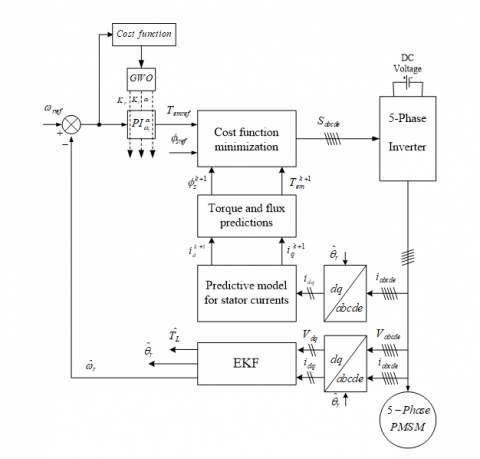Figure 2. Sensorless PDTC scheme using PIᵅ-GWO controller for a five-phase PMSM drive based on EKF observer

5.1.1 Predictive model for stator currents

From the five-phase PMSM model described in Section 2, the stator current dynamics can be given by:

\left\{ \begin{align}& \frac{d{{i}_{d}}}{dt}=-\frac{{{R}_{s}}}{{{L}_{d}}}{{i}_{d}}+\frac{{{L}_{q}}}{{{L}_{d}}}p{{\omega }_{r}}{{i}_{q}}+\frac{{{V}_{d}}}{{{L}_{d}}} \\ & \frac{d{{i}_{q}}}{dt}=-\frac{{{R}_{s}}}{{{L}_{q}}}{{i}_{q}}-\frac{{{L}_{d}}}{{{L}_{q}}}p{{\omega }_{r}}{{i}_{d}}-\frac{{{\phi }_{f}}}{{{L}_{q}}}p{{\omega }_{r}}+\frac{{{V}_{q}}}{{{L}_{q}}} \\\end{align} \right.   (13)

To predict the next step value, the forward Euler discretization is considered by adopting the following approximation:

$\frac{dx}{dt}\approx \frac{{{x}^{k+1}}-{{x}^{k}}}{{{T}_{s}}}$      (14)

where, Ts is the sampling period.

Using Eqns. (13) and (14), the dq-axes components of stator current can be predicted as:

$\left\{\begin{array}{l}i_{d}^{k+1}=i_{d}^{k}+\frac{1}{L_{d}}\left(-R_{s} i_{d}^{k}+p \omega_{r}^{k} L_{q} i_{q}^{k}+V_{d}^{k}\right) T_{s} \\ i_{q}^{k+1}=i_{q}^{k}+\frac{1}{L_{q}}\left(-R_{s} i_{q}^{k}+p \omega_{r}^{k} L_{d} i_{d}^{k}-p \omega_{r}^{k} \phi_{f}+V_{q}^{k}\right) T_{s}\end{array}\right.$  (15)

5.1.2 Torque and stator flux prediction

According to Eqns. (2) and (15), the prediction of the stator flux components at the next sampling instant can be expressed as follows:

\left\{ \begin{align}& \phi _{d}^{k+1}={{L}_{d}}i_{d}^{k+1}+{{\phi }_{f}} \\& \phi _{q}^{k+1}={{L}_{q}}i_{q}^{k+1} \\\end{align} \right.      (16)

So, the stator flux amplitude is given by:

$\phi _{s}^{k+1}=\sqrt{{{\left( \phi _{d}^{k+1} \right)}^{2}}+{{\left( \phi _{q}^{k+1} \right)}^{2}}}$       (17)

Based on Eq. (3), with the predicted stator flux Eq. (16) and predicted currents Eq. (15), the electromagnetic torque can be predicted as:

${{T}_{em}}=\frac{5}{2}p\left( \phi _{d}^{k+1}i_{q}^{k+1}-\phi _{q}^{k+1}i_{d}^{k+1} \right)$   (18)

5.1.3 Cost function minimization

The cost function is chosen such that both torque and stator flux at the end of the cycle will be as close as possible to their reference values. One way to define the cost function is as follows:

$F=\left| {{T}_{emref}}-T_{em}^{k+1} \right|+{{k}_{\phi }}\left| {{\phi }_{sref}}-\phi _{s}^{k+1} \right|$      (19)

where, $k_{\phi}$  is a weighting factor.

This function will be calculated for each switching vector $V_{s}^{k} \in\left\{V_{1}, V_{2}, \ldots, V_{10}\right\}$ , and the vector that minimizes (19) will be chosen.

5.2 Fractional order PI controller

5.2.1 Basic definitions of fractional calculus

The fractional differ-integral operators symbolized by ${ }_{a} D_{t}^{\alpha} f(t)$ that is considered as a generalization of integration and differentiation operators of a non-integer order. There are several definitions for this notion in the literature, the most used are :

The Riemann-Liouville (RL) definition:

${}_{a}D_{t}^{\alpha }f(t)=\frac{1}{\Gamma \left( m-\alpha \right)}{{\left( \frac{d}{dt} \right)}^{m}}\int_{a}^{t}{\frac{f(\tau )}{{{\left( t-\tau \right)}^{1-\left( m-\alpha \right)}}}d\tau }$    (20)

The Caputo’s definition:

${}_{a}D_{t}^{\alpha }f(t)=\frac{1}{\Gamma \left( m-\alpha \right)}\int_{a}^{t}{\frac{{{f}^{m}}(\tau )}{{{\left( t-\tau \right)}^{1-\left( m-\alpha \right)}}}d\tau }$      (21)

where, $m-1<\alpha<m$ and $\Gamma(\cdot)$  is the well-known Euler’s gamma function, and its definition is:

$\Gamma \left( x \right)=\int_{0}^{\infty }{{{e}^{-t}}{{t}^{(x-1)}}}dt,x>0$     (22)

Otherwise, Grunwald-Letnikov (GL) suggested reformulating the definition of the fractional order differ-integral as follows:

${}_{a}D_{t}^{\alpha }f(t)={}_{h}\operatorname{l}{{}_{0}}\frac{1}{{{h}^{\alpha }}}\sum\limits_{k=0}^{\left( t-a \right)/h}{{{\left( -1 \right)}^{k}}\left( \begin{matrix} \alpha \\ k \\\end{matrix} \right)}\,f\left( t-kh \right)$   (23)

Due to the complexity of the numerical simulation of a fractional differential equation compared to ordinary differential equation, so the Laplace transform method is often used as a tool to solve emerging problems in engineering fields. [50, 51].

The Laplace transform of the fractional order derivative given previously by (RL) definition is as follows [26, 51]:

${{e}^{st}}{}_{0}D_{t}^{\alpha }f(t)={{s}^{\alpha }}F\left( s \right)-{{\sum\limits_{k=0}^{m-1}{{}_{0}D_{t}^{\alpha -k-1}f(t)}}_{t=0}}$ (24)

where, s=jw denotes the Laplace operator. For zero initial conditions, the Laplace transform of fractional derivative is reduced to [26, 52]:

$L\left( {}_{0}D_{t}^{\alpha }f(t) \right)={{s}^{\alpha }}F\left( s \right)$    (25)

The fractional order element $G(s)=s^{\alpha}, \alpha \in R^{+}$  can be approximated with Oustaloup’s filter , which is relied on the approximation of a rational function of the form:

$\hat{G}(s)={{K}^{'}}\prod\limits_{k=-{{N}^{'}}}^{{{N}^{'}}}{\frac{s+w_{k}^{'}}{s+{{w}_{k}}}}$      (26)

Determine the zeros, poles, and gain of this function are given by using the following formulas:

\left\{ \begin{align} & w_{k}^{'}={{w}_{b}}{{\left( \frac{{{w}_{h}}}{{{w}_{b}}} \right)}^{{\left( k+{{N}^{'}}+0.5\left( 1-\alpha \right) \right)}/{\left( 2{{N}^{'}}+1 \right)}\;}} \\& w_{k}^{{}}={{w}_{b}}{{\left( \frac{{{w}_{h}}}{{{w}_{b}}} \right)}^{{\left( k+{{N}^{'}}+0.5(1+\alpha ) \right)}/{\left( 2{{N}^{'}}+1 \right)}\;}} \\& {{K}^{'}}=w_{h}^{\alpha } \\\end{align} \right.      (27)

The number $\left(2 N^{\prime}+1\right)$  is the order of the filter, wb and wh are the low and high transient-frequencies, respectively.

Lemma 1. Consider the following autonomous system :

${}_{0}D_{t}^{\alpha }=Cy(t),\text{ }y(0)={{y}_{0}}$   (28)

where, $y \in R^{n}, C=\left(c_{i j}\right) \in R^{n \times n}, 0<\alpha<1$, is asymptotically stable if the following condition is verified (see Figure 3):

$\left| \arg (eig(A)) \right|>\alpha \frac{\pi }{2}$      (29)

in which each component of the states decays towards 0 like $t^{-\alpha}$

## ff3.pngFigure 3. Stable domain of fractional order system in $S^{\alpha}$  plane

5.2.2 Speed fractional controller

The structure of the speed fractional controller is illustrated in Figure 4; its output can be determined by the following formula:

${{T}_{emref}}={{k}_{p}}e(t)+{{k}_{i}}D_{t}^{-\alpha }e(t)$     (30)

where, $e(t)=\omega_{m e f}-\hat{\omega}_{r}$  is speed error.

The transfer function of this controller is given by:

$e(t)=\omega_{n e f}-\hat{\omega}_{r}$     (31)

The fractional order $P I^{\alpha}$  controller can improve the control performance and give good results thanks to its extra real parameter $\alpha$  implying a greater flexibility.

Note that, when choosing the parameter $\alpha=1$ , classical PI controller is obtained.

## ff4.png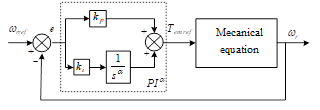Figure 4. Structure of fractional order $P I^{\alpha}$  controller

5.3 GWO-based parameters tuning of the PIᵅ controller

The fractional order PI controller has three parameters to be tuned $k_{p}, k_{i}, \alpha$. To determine these parameters, one of the evolutionary algorithms can be used, known as the gray wolf algorithm that is recently invented, to adjust and obtain the optimal values by minimizing the Integral Time Absolute Error (ITAE) criteria, that is given by Eq. (32) as follows:

$F=\int\limits_{0}^{t}{t\left| e\left( t \right) \right|}dt$       (32)

GWO algorithm is a new meta-heuristic optimization method, which is suggested by Mirjalili et al. . It emulates the leadership hierarchy and hunting behavior of grey wolves in nature. To design and emulate this algorithm, it requires modeling for these two social behaviors of grey wolves’ pack (social hierarchy and hunting technique), which is as follows [33-41].

## ff5.pngFigure 5. Social hierarchy of grey wolf

5.3.1 Social hierarchy

For modeling the social hierarchy of grey wolves in the GWO algorithm, alpha $\alpha$  represents the best solution, Thence, beta $\beta$  and delta $\delta$  represent the second and third best solutions respectively, and omega $\omega$  represents the rest of the solutions. In the GWO the hunting (optimization) is led by $\alpha, \beta,$ and $\delta$ . The $\omega$  wolves pursue them. The social hierarchy of the grey wolf is shown in Figure 5.

5.3.2 Encircling prey

Pending the hunting, the grey wolves encircle prey, encircling behavior can be represented by the following equations:

$\overrightarrow{M}=\left| \overrightarrow{C}\,\overrightarrow{P}(t)-\overrightarrow{W}(t) \right|$    (33)

$\overrightarrow{W}(t+1)=\overrightarrow{P}(t)-\overrightarrow{A}\,\overrightarrow{D}$   (34)

where, t indicates the current iteration, $\vec{A}$  and $\bar{C}$  are coefficient vectors, $\vec{P}$  represents the position vector of the prey, and $\vec{W}$  is considered the position vector of a grey wolf. The vectors $\vec{A}$  and $\bar{C}$ are computed by:

$\overrightarrow{A}=2\overrightarrow{\,a}\,{{\overrightarrow{r}}_{_{1}}}-\overrightarrow{a}$     (35)

$\overrightarrow{C}=2.{{\overrightarrow{r}}_{_{2}}}$     (36)

where, components of $a$ are linearly decreased from 2 to 0 over the course of iterations, and r1 and r2 are random vectors in [0, 1]. Note that the random r1 and r2 vectors let the grey wolf select any positions in Figure 6. Therefore, a grey wolf can be placed in each random position around the prey that is calculated by using Eqns. (33) and (34).

## ff6.png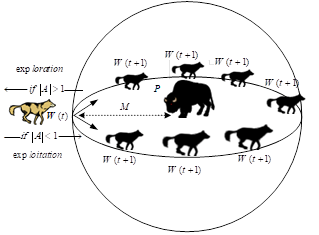Figure 6. Position updating mechanism of search agents and effects of A on it.

5.3.3 Hunting

In order to model hunting behavior, assume that the alpha α, beta β and delta δ are well aware of the Likely site of prey. The first three best solutions are memorized and the other agents are obliged to update their positions according to the position of the best search agents as shown in the following equations.

\begin{align} & {{\overrightarrow{M}}_{\alpha }}=\left| {{\overrightarrow{C}}_{1}}\,{{\overrightarrow{P}}_{\alpha }}-\overrightarrow{W} \right| \\& {{\overrightarrow{M}}_{\beta }}=\left| {{\overrightarrow{C}}_{2}}\,{{\overrightarrow{P}}_{\beta }}-\overrightarrow{W} \right| \\& {{\overrightarrow{M}}_{\delta }}=\left| {{\overrightarrow{C}}_{3}}\,{{\overrightarrow{P}}_{\delta }}-\overrightarrow{W} \right| \\\end{align}     (37)

\begin{align}& {{\overrightarrow{W}}_{1}}={{\overrightarrow{P}}_{\alpha }}-{{\overrightarrow{A}}_{1}}\,{{\overrightarrow{M}}_{\alpha }} \\& {{\overrightarrow{W}}_{2}}={{\overrightarrow{P}}_{\beta }}-{{\overrightarrow{A}}_{2}}\,{{\overrightarrow{M}}_{\beta }} \\& {{\overrightarrow{W}}_{3}}={{\overrightarrow{P}}_{\delta }}-{{\overrightarrow{A}}_{3}}\,{{\overrightarrow{M}}_{\delta }} \\\end{align}   (38)

$\overrightarrow{W}(t+1)=\frac{{{\overrightarrow{W}}_{1}}+{{\overrightarrow{W}}_{2}}+{{\overrightarrow{W}}_{3}}}{3}$   (39)

The search agent position updating process is shown in Figure 7. The final position (solution) is inside a circle that is specified based on the positions of α, β and δ in the decision space. In other words, α, β and δ estimate the positions of prey and other wolves and then update their new positions, randomly around the prey.

5.3.4 Attacking prey (exploitation)

Hunting ends with attack on prey if it stops. The vector A is a random value in interval [-2a, 2a], if |A|<1, the wolves attack the prey, that represents an exploitation operation.

## ff7.png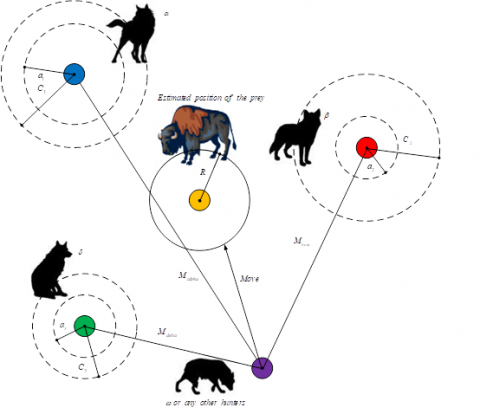Figure 7. Position updating in GWO

5.3.5 Search for prey (exploration)

Depending on the position α, β and δ, the exploration operation in GWO is used, that diverges from each other to search for prey and converges to attack prey. If $|A|>1$, as shown in Figure 6, the wolves are obliged to diverge from the prey to locate more appropriate prey.

Implementation of GWO is as follows.

 Begin Step 1 : Initialize the position $W_{i}(i=1,2, \ldots, n)$  of each search    agent   Initialize the value of $a_{,} A,$ and $C$ Step 2 : For each $W_{i}$  do Compute the fitness value of each search agent by Eq. (32) End for Save the first three best solutions as $W_{\alpha}$, $W_{\beta}$, and $W_{\delta \mid}$ Step 3 : While (i
6. Simulation Results

In this section, to evaluate the performance and effectiveness of the proposed control using EKF observer, a series of numerical simulations is carried out. Parameters of five-phase PMSM are given in Table 2.

Table 2. Five-phase PMSM parameters

 p Ld Lq $\phi_{f}$ J Rs f 2 8mH 8.5mH 0.175Wb 0.004kgm2 1 $\Omega$ 0

The EKF parameters are chosen as follows:

$\left[ P \right]=\left[ \begin{matrix} {{10}^{-3}} & 0 & 0 & 0 & 0 \\ 0 & {{10}^{-3}} & 0 & 0 & 0 \\0 & 0 & {{10}^{-1}} & 0 & 0 \\ 0 & 0 & 0 & 10 & 0 \\ 0 & 0 & 0 & 0 & {{10}^{-4}} \\\end{matrix} \right]$ ,

$\left[ R \right]=\left[ \begin{matrix}0.02 & 0 \\0 & 0.022 \\\end{matrix} \right]$

$\left[ Q \right]=\left[ \begin{matrix}{{10}^{-6}} & 0 & 0 & 0 & 0 \\0 & {{10}^{-6}} & 0 & 0 & 0 \\0 & 0 & {{10}^{-5}} & 0 & 0 \\0 & 0 & 0 & {{10}^{-5}} & 0 \\0 & 0 & 0 & 0 & {{10}^{-5}} \\\end{matrix} \right]$

The population size of GWO algorithm is set to 30 particles, and the maximum number of iteration J is set to 30 iterations.

The five-phase PMSM is accelerated from standstill to reference speed (100 rad/s). The system is started with full load torque (TL=5Nm). Afterwards, a step variation of the load torque (TL=0Nm) is applied at time t = 1 s. Then a sudden reversion in the speed command from (100 rad/s) to (–100 rad/s) is introduced at 1.5 s.

The dynamic responses of speed, electromagnetic torque, stator flux, and estimated load torque are shown in Figures 8 and 9 for C-DTC and PDTC using PI controller based on EKF observer, and in Figures 10 and 11 for PDTC with PI-GWO and $P I^{\alpha}-G W O$  controllers based also on EKF observer.

From Figures 8(a) and 9(a), the speed follows its reference value. Disturbance caused by load torque variation is rejected thanks to the intervention of speed controller, which guarantees that the speed follows its suitable reference. When comparing between the C-DTC and PDTC strategies based on PI controller, there is any noticeable difference in term of speed tracking. In addition, it can be observed also that the estimated speed shows good reference tracking and coincides with the actual one in all speed regions. This confirms the robustness and efficacy of the used observer.

The electromagnetic torque curves generated by the five-phase PMSM are shown in Figures 8(b) and 9(b) for both strategies, C-DTC and PDTC based on PI controller. Note that the proposed control can minimize the torque ripples, which reflects the accurate choice of the optimal switching vectors.

From Figures 8(c) and 9(c), note that the stator flux has a fast and good reference tracking without any influence by the load variation, this implies that the decoupling between the stator flux and the electromagnetic torque is maintained. In addition, the proposed control can decrease the stator flux ripples compared to C-DTC.

Figures 8(d) and 9(d) illustrate the load torque estimation for both strategies. It can see that the estimated value is quite close to the actual value.

## a.png(a)

## b.png(b)

## c.png(c)

## d.png(d)

Figure 8. Dynamic responses of the five-phase PMSM using EKF controlled by C-DTC with PI controller

Simulation results of PDTC using PI-GWO and PI-GWO controllers are shown in Figures 10 and 11, respectively. These results show that the use of PI-GWO controller increases and enhances the robustness of the control against speed and load changes. This improves the efficiency and performance of control. With regard to the electromagnetic torque and the stator flux responses, which are illustrated in Figures 10(b), 11(b) and Figures 10(c), 11(c), respectively, there are no noticeable differences between the two control approaches, in which the decoupling between the electromagnetic torque and the stator flux is fully maintained.

The load torque estimation is illustrated in Figures 10(d) and 11(d) for both strategies. Note that the estimated value is very close to the actual value.

Figures 12 shows the cost function evolution during the optimization process with GWO; after 30 iterations the cost function converges to zero, where the minimum value of the cost function determines the best set of design parameters. This reflects that the best response is obtained by the usage of fractional order PI controller compared to classical PI controller.

## aa.png(a)

## bb.png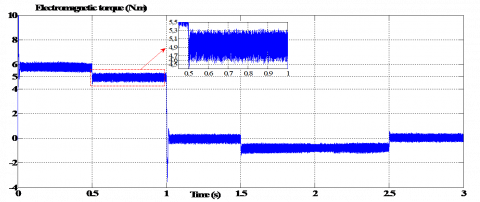(b)

## cc.png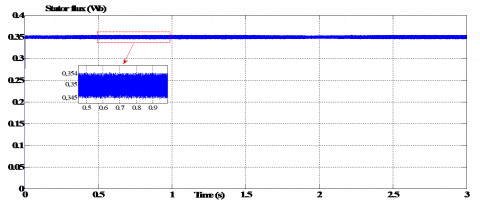(c)

## dd.png(d)

Figure 9. Dynamic responses of the five-phase PMSM using EKF controlled by PDTC with PI controller

Comparative study

In order to check the efficacy of the proposed controller in tracking the reference value compared to classical PI controller and classical PI controller tuned by GWO for both C-DTC and PDTC, four well known performance criteria are used. These are integral of the absolute value of the error (IAE), integral of the time multiplied by the absolute value of the error (ITAE), integral of square error (ISE), and integral of the time multiplied by square error (ITSE). The values that are obtained for each criterion at the end of simulation time (3 s) are illustrated in Figure 13. Note that the proposed controller gives the lowest value to all the above criteria. This result confirms the superiority of the fractional order PI controller tuned by GWO compared to the aforementioned controllers in term of tracking performance.

From Figure 14, it can be seen that PDTC using fractional order PI controller tuned by GWO, under the same operating conditions, has smaller ripples compared to C-DTC using PI controller and PDTC using both PI controller and PI-GWO controller. This result confirms once again the superiority of the proposed control in term of ripples reduction.

## a.png(a)

## b.png## b.png(b)

## c.png(c)

## d.png(d)

Figure 10. Dynamic responses of the five-phase PMSM using EKF controlled by PDTC with PI-GWO controller

## a.png(a)

## b.png(b)

## c.png(c)

## d.png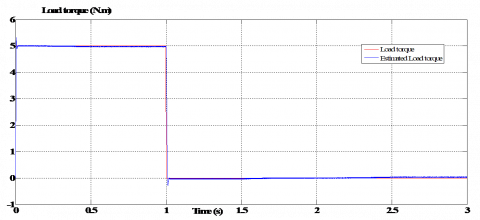(d)

Figure 11. Dynamic responses of the five-phase PMSM using EKF controlled by PDTC with PI-GWO controller

## 12.pngFigure 12. Evolution of the cost function versus iteration for PDTC based on EKF observer: Using PI-GWO and PI-GWO controllers

7. Conclusion

In this paper, a PDTC and fractional order PI controller tuned by GWO are associated together in order to improve the performance of the conventional DTC based on PI controller for a five-phase PMSM drive. According to the simulation results, the proposed control outperforms its counterpart conventional DTC based on PI controller under various operating conditions in term of tracking performance, reduced torque and stator flux ripples, and robustness against external disturbance.

Furthermore, the combination of the control scheme with an observer increases the reliability and decreases the size, and cost of the drive system. The performances and effectiveness of EKF are verified. Simulation results show the ability of the proposed observer to ensure good estimates.

## 13.pngFigure 13. Performance criteria values of speed error for C-DTC and PDTC based on EKF observer: Using PI, PI-GWO and PI-GWO controllers

## 14.png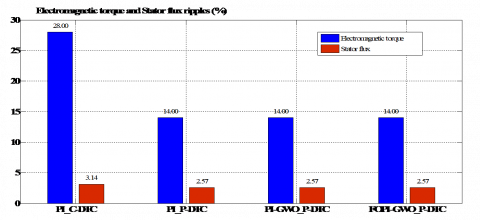Figure 14. Electromagnetic torque and stator flux ripples for C-DTC and PDTC based on EKF observer: Using PI, PI-GWO and PI-GWO controllers

References

 Difi, D., Halbaoui, K., Boukhetala, D. (2019). Hybrid control of five-phase permanent magnet synchronous machine using space vector modulation. Turkish Journal of Electrical Engineering & Computer Sciences, 27(2): 921-938. https://doi.org/10.3906/elk-1805-193

 Zhou, Y., Yan, Z., Duan, Q., Wang, L., Wu, X. (2019). Direct torque control strategy of five-phase PMSM with load capacity enhancement. IET Power Electronics, 12(3): 598-606. https://doi.org/10.1049/iet-pel.2018.5203

 Levi, E., Barrero, F., Duran, M.J. (2016). Multiphase machines and drives-Revisited. IEEE Trans. Ind. Electron, 63(1): 429-432. https://doi.org/10.1109/TIE.2015.2493510

 Mohammad, A., Parsa, L. (2015). Global fault-tolerant control technique for multiphase permanent-magnet machines. IEEE Transactions on Industry Applications, 51(1): 178-186. https://doi.org/10.1109/TIA.2014.2326084

 Payami, S., Behera, R.K., Yu, X., Gao, M. (2017). An improved DTC technique for low speed operation of a five-phase induction motor. IEEE Transactions on Industrial Electronics, 64(5): 3513-3523. https://doi.org/10.1109/TIE.2017.2652397

 Hamdi, E., Ramzi, T., Atif, I., Mimouni, M.F. (2018). Adaptive direct torque control using Luenberger-sliding mode observer for online stator resistance estimation for five-phase induction motor drives. Electrical Engineering, 100: 1639-1649. https://doi.org/10.1007/s00202-017-0639-7

 Khaldi, B.S., Abu-Rub, H., Iqba, A., Kennel, R., Mahmoudi, M.O., Boukhetala, D. (2011). Sensorless direct torque control of five-phase induction motors drives. IECON, 37th Annual Conference, Melbourne, VIC, pp. 3501-3506. https://doi.org/10.1109/IECON.2011.6119875

 Foo, G., Rahman, M.F. (2010). Direct torque control of an IPM-synchronous motor drive at very low speed using a sliding mode stator flux observer. IEEE Transactions on Power Electronics, 25(4): 933-942. https://doi.org/10.1109/TPEL.2009.2036354

 Parsa, L., Toliyat, H.A. (2007). Sensorless direct torque control of five-phase interior permanent-magnet motor drives. IEEE Transactions on Industry Applications, 43(4): 952-959. https://doi.org/10.1109/TIA.2007.900444

 Barika, S.K., Jaladi, K.K. (2016). Five-phase induction motor DTC-SVM scheme with PI controller and ANN controller. Procedia Tech., 25: 816-823. https://doi.org/10.1016/j.protcy.2016.08.184

 Ahriche, A., Kidouche, M., Mekhilef, S. (2014). Robust sensorless sliding mode flux observer for DTC-SVM-based drive with inverter nonlinearity compensation. Journal of Power Electronics, 14(1): 125-134. https://doi.org/10.6113/JPE.2014.14.1.125

 Chikh, K., Saad, A., Khafallah, M., Yousfi, D. (2011). A novel drive implementation for PMSM by using direct torque control with space vector modulation. Canadian Journal on Electrical and Electronics Engineering, 2(8): 400-408.

 Zhang, Z., Tang, R., Bai, B., Xie, D. (2010). Novel direct torque control based on space vector modulation with adaptive stator flux observer for induction motors. IEEE Trans. Magnet, 46(8): 3133-3136. https://doi.org/10.1109/TMAG.2010.2051142

 Cho, Y., Bak, Y., Lee, K.B. (2018). Torque-ripple reduction and fast torque response strategy for predictive torque control of induction motors. IEEE Trans. Power Electron., 33(3): 2458-2470. https://doi.org/10.1109/TPEL.2017.2699187

 Mesloub, H., Benchouia, M.T., Goléa, A., Goléa, N., Benbouzid, M.E.H. (2016). Predictive DTC schemes with PI regulator and particle swarm optimization for PMSM drive: Comparative simulation and experimental study. The International Journal of Advanced Manufacturing Technology, 86(9): 3123-3134. https://doi.org/10.1007/s00170-016-8406-x

 Wang, F., Li, S., Mei, X., Xie, W., Rodríguez, J. (2015). Model-based predictive direct control strategies for electrical drives: an experimental evaluation of PTC and PCC methods. IEEE Transactions on Industrial Informatics, 11(3): 671-681. https://doi.org/10.1109/TII.2015.2423154

 Riveros, A.J., Barrero, F., Levi, E., Durán, M.J., Toral, S., Jone, M. (2013). Variable-speed five-phase induction motor drive based on predictive torque control. IEEE Transactions on Industrial Electronics, 60(8): 2957-2968. https://doi.org/10.1109/TIE.2012.2198034

 Geyer, T., Papafotiou, G., Morari, M. (2009). Model predictive direct torque control-part I: Concept, algorithm, and analysis. IEEE Transactions on Industrial Electronics, 56(6): 1894-1905. https://doi.org/10.1109/TIE.2008.2007030

 Pacas, M., Weber, J. (2005). Predictive direct torque control for the PM synchronous machine. IEEE Transactions on Industrial Electronics, 52(5): 1350-1356. https://doi.org/10.1109/TIE.2005.855662

 Alkorta, P., Barambones, O., Cortajarena, J.A., Zubizarrreta, A. (2014). Efficient multivariable generalized predictive control for sensorless induction motor drives. IEEE Trans. Ind. Electron., 61(9): 5126-5134. https://doi.org/10.1109/TIE.2013.2281172

 Puangdownreong, D. (2019). Fractional order PID controller design for DC motor speed control system via flower pollination algorithm. Transactions on Electrical Engineering, 17(1). https://doi.org/10.37936/ecti-eec.2019171.215368

 Saleem, A., Soliman, H., Al-Ratrout, S., Mesbah, M. (2018). Design of a fractional order PID controller with application to an induction motor drive. Turkish Journal of Electrical Engineering & Computer Sciences, 26(5): 2768-2778. https://doi.org/10.3906/elk-1712-183

 Mohan, V., Chhabra, H., Rani, A., Singh, V. (2018). Robust self-tuning fractional order PID controller dedicated to non-linear dynamic system. Journal of Process Control, 34(3): 1467-1478. https://doi.org/10.3233/JIFS-169442

 Bouarroudj, N., Boukhetala, D., Boudjema, F. (2016). Sliding-mode controller based on fractional order calculus for a class of nonlinear systems. International Journal of Electrical and Computer Engineering, 6(5): 2239-2250. https://doi.org/10.11591/ijece.v6i5.pp2239-2250

 Bouarroudj, N., Boukhetala, D., Boudjema, F. (2015). A hybrid fuzzy fractional order PID sliding mode controller design using PSO algorithm for interconnected nonlinear systems. Control Engineering and Applied Informatics, 17(1): 41-51.

 Podlubny, I. (1999). Fractional-order systems and  controllers. IEEE Trans. Automatic Control, 44(1): 208-214. https://doi.org/10.1109/9.739144

 Valério, D., Costa, J. (2010). A review of tuning methods for fractional PIDs. Proc. 4th IFAC Workshop Fract. Differ. Appl., Badajoz, Spain, pp. 1-5.

 Zhong, J., Li, L. (2015). Tuning fractional-order  controllers for a solid-core magnetic bearing system. IEEE Trans. Control Sys. Tech., 23(4): 1648-1656. https://doi.org/10.1109/TCST.2014.2382642

 Valério, D., Costa, J. (2006). Tuning of fractional PID controllers with Ziegler–Nichols-type rules. Signal Process., 86(10): 2771-2784. https://doi.org/10.1016/j.sigpro.2006.02.020

 Necaibia, A., Ladaci, S. (2014). Self-tuning fractional order  controller based on extremum seeking approach. International Journal of Automation and Control (IJAAC), 8(2): 99-121. https://doi.org/10.1504/IJAAC.2014.063361

 Bingul, Z., Karahan, O. (2012). Fractional PID controllers tuned by evolutionary algorithms for robot trajectory control. Turk. J. Elec. Eng. Comp. Sci., 20(1): 1123-1126. https://doi.org/10.3906/elk-1102-1011

 Mirjalili, S., Mirjalili, S.M., Lewi, A. (2014). Grey wolf optimizer. Advances in Engineering Software, 69: 46-61. https://doi.org/10.1016/j.advengsoft.2013.12.007

 Mirjalili, S. (2015). How effective is the grey wolf optimizer in training multi-layer perceptrons. Applied Intelligence, 43(1): 150-161. https://doi.org/10.1007/s10489-014-0645-7

 Song, X., Tang, L., Zhao, S.T., Zhang, X.Q., Li, L., Huang, J.Q., Cai, W. (2015). Grey wolf optimizer for parameter estimation in surface waves. Soil. Dynamics and Earth quake Engineering, 75: 147-157. https://doi.org/10.1016/j.soildyn.2015.04.004

 Komaki, G.M., Kayvanfar, V. (2015). Grey wolf optimizer algorithm for the two-stage assembly flow shop scheduling problem with release time. Journal of Computational Science, 8: 109-120. https://doi.org/10.1016/j.jocs.2015.03.011

 Guha, D., Roy, P.K., Banerjee, S. (2016). Load frequency control of interconnected power system using grey wolf optimization. Swarm and Evolutionary Computation, 27: 97-115. https://doi.org/10.1016/j.swevo.2015.10.004

 Sulaiman, M.H., Mustaffa, Z., Mohamed, M.R., Aliman, O. (2015). Using the gray wolf optimizer for solving optimal reactive power dispatch problem. Applied Soft Computing, 32: 286-292. https://doi.org/10.1016/j.asoc.2015.03.041

 Noshadi, A., Shi, J., Lee, W.S., Shi, P., Kalam, A. (2016). Optimal PID-type fuzzy logic controller for a multi-input multi-output active magnetic bearing system. Neural Computing and Applications, 27: 2031-2046. https://doi.org/10.1007/s00521-015-1996-7

 de Moura Oliveira, P.B., Freire, H., Solteiro Pires, E.J. (2016). Grey wolf optimization for PID controller Design with prescribed robustness margins. Soft Computing, 20(11): 4243-4255. https://doi.org/10.1007/s00500-016-2291-y

 Mohanty, S., Subudhi, B., Ray, P.K. (2017). A grey wolf assisted Perturb & Observe MPPT algorithm for a PV system. IEEE Trans. Energy Conversion, 32(1): 340-347. https://doi.org/10.1109/TEC.2016.2633722

 Mohammed, H.Q., Hany, M.H., Saad, A. (2018). Augmented grey wolf optimizer for grid-connected PMSG-based wind energy conversion systems. Applied Soft Computing, 69: 504-515. https://doi.org/10.1016/j.asoc.2018.05.006

 Ammar, A., Bourek, A., Benakcha, A. (2017). Robust SVM-Direct torque control of induction motor based on sliding mode controller and sliding mode observer. Frontiers in Energy, 1-14. https://doi.org/10.1007/s11708-017-0444-z

 Messaoudi, M., Sbita, L. (2017). Sensorless direct torque and flux control of induction motor based on MRAS and Luenberger observer. IEEE. Inter. Conf. GECS, Hammamet, Tunisia. https://doi.org/10.1109/GECS.2017.8066224

 Aissa, A., Mokhtari, B. (2012). Extended Kalman filter for speed sensorless direct torque control of a PMSM drive based stator resistance estimator. Journal of Electrical and Control Engineering, 2(6): 33-39.

 Zhang, Y., Cheng, X.F. (2016). Sensorless control of permanent magnet synchronous motors and EKF parameter tuning research. Mathematical Problems in Engineering, 8: 1-12. https://doi.org/10.1155/2016/3916231

 Bounasla, N., Barkat, S., Benyoussef, E., Tounsi, K. (2016). Sensorless sliding mode control of a five-phase PMSM using extended kalman filter. 8th ICMIC, Algeria, pp. 97-102. https://doi.org/10.1109/ICMIC.2016.7804280

 Warsame, A.H., Gowda, M., Cofie, P., Fuller, J. (2014). Design of a speed controller using extended kalman filter for PMSM. IEEE 57th Inter. Midwest Symp. Circui. Sys., USA, pp. 1101-1104. https://doi.org/10.1109/MWSCAS.2014.6908611

 Anwer, A.M.O., Omar, F.A., Bakir, H., Kulaksiz, A.A. (2020). Sensorless control of a PMSM drive using EKF for wide speed range supplied by MPPT based solar PV system. Elektron. Elektrot., 26(1): 32-39. https://doi.org/10.5755/j01.eie.26.1.25308

 Mukhtar, A. (2010). High Performance AC Drives Modelling Analysis and Control. Springer-Verlag London.

 Miler, K.S., Ross, R. (1993). An Introduction to The Fractional Calculus and Fractional Differential Equations. Wiley, New York.

 Oldham, K., Spanier, J. (1974). The Fractional Calculus: Theory and Application of Differentiation and Integration to Arbitrary Order. Wiley, New York.

 Ortigueira, M.D., Trujillo, J.J. (2009). Generalized GL fractional derivative and its Laplace and Fourier transform. Inter. DETC/CIE, San Diego, California, USA, pp. 1227-1231. https://doi.org/10.1115/DETC2009-87238

 Oustaloup, A., Levron, F., Mathieu, B.M., Nanot, F.M. (2000). Frequency-band complex noninteger differentiator: Characterization and synthesis. IEEE Trans. Circuits Syst. I. Fundam. Theory Appl., 47(1): 25-39. https://doi.org/10.1109/81.817385

 Hamouda, N., Babes, B., Hamouda, C., Kahla, S., Ellinger, T., Petzoldt, J. (2020). Optimal tuning of fractional order PID controller for wire feeder system using ant colony optimization. Journal Européen des Systèmes Automatisés, 53(2): 157-166. https://doi.org/10.18280/jesa.530201